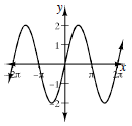### Home > INT3 > Chapter 10 > Lesson 10.3.1 > Problem10-147

10-147.

Graph two complete cycles of each function.

1. $y = - 2 \operatorname { cos } ( x + \frac { \pi } { 2 } )$

What is the amplitude? Where is the locator point? What is the orientation? What is the period?1. $y = \operatorname { sin } ( x - \frac { \pi } { 2 } )$

See part (a).